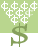Rags to Riches: Answer questions in a quest for fame and fortune.# CCA 3.1.1 - Multiplication Properties of Exponents (M.L. 8.1)

Please understand that 3^2 means 3 squared, or 9. Good luck playing this game!

The exponent "PRODUCT RULE" tells us that, when multiplying two powers that have the same base, you can add the exponents.

The "QUOTIENT RULE" tells us that we can divide two powers with the same base by subtracting the exponents.

The "POWER of a POWER" Rule tells us that to raise a power to a power, just multiply the exponents.

"POWER OF A PRODUCT Rule": Definition: \$ (xy)^8 = x^8y^8\$This activity was created by a Quia Web subscriber. Learn more about QuiaCreate your own activities